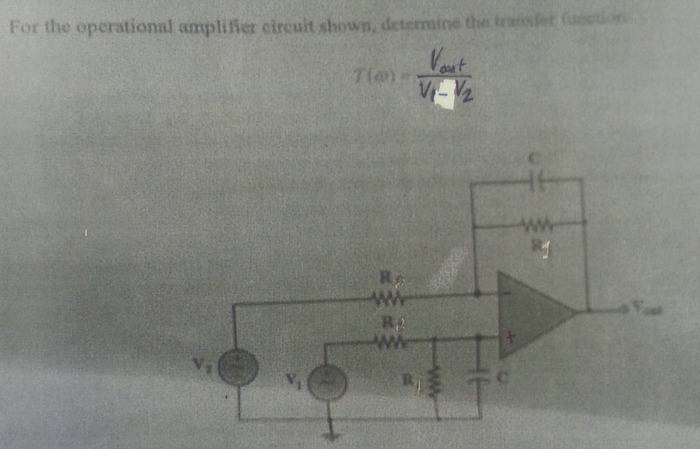# Op amp transfer function determination (need help)

OP warned about not using the homework template
Hey guys, need help with a problem here.

Sorry for the quality of the image.I understand that above the op-amp, is the active low pass filter. So if I were to figure that out with respect to V2 and Vout, it would just be

Vout/Vs = -(Zf/Zs)

where

Zf = (Rf/(1+jwCfRf))
Zs = Rs

But I don't understand how the bottom setup comes into the equation. I realize it must also be a filter and should be solved wrt V1.

And I am asked to find the transfer function Vout/V1-V2.

All help would be greatly appreciated.

## Answers and Replies

Because you have two input sources you can derive two different transfer functions (one inverting and the other one non-inverting).
And - as a second step - it should not be a problem to substract both functions Vout=Vout1-Vout2.